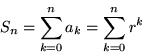The Sum of A Geometric Series of Lengths.

We consider a sequence of lengths where the ratio of each length to the previous length is a constant.
Examples:

1. If the ratio is 1/2 and the first length has a measurement of 4, then the sequence is 4,2,1,1/2,1/4,1/8,1/16,...

2. If the ratio is 1/5 and the first length has a measurement of 25, then the sequence is 25,5,1,1/5,1/25,1/125,1/625,...

3. If the ratio is 2/3 and the first length has a measurement of 9, then the sequence is 9,6,4,8/3,16/9,32/27,64/81,128/243,...

4. If the ratio is 4 and the first length has a measurement of 3, then the sequence is 3,12,48,152,...

5. If the ratio is 4/3 and the first length has a measurement of 3, then the sequence is 3, 4, 16/3, 64/9, 256/27, 1024/81,...

6. If the ratio is r and the first length has a measurement of 1, then the sequence is 1, r, r2, r3, r4, r5,...

A sequence of this type is called a geometric sequence.
Suppose we add the terms of a geometric sequence:

Example: 4 + 2+1 + 1/2 +1/4 + 1/8 +... = 7 + "1" = 8

BUT ... what about the other sequences?

Consider  the general  sequence 1, r, r2, r3, r4, r5,... and the sum of the first n terms,.

Then  for any r where 0<r<1 and a large  value of n, S n is close to 1/(1-r).

Here is a visual argument to justify the previous statement:
In the figure below, consider the left  most square to have side of length 1.

There are several similar squares with the ratios of the sides being r/1 in the figure where 0<r<1.

Using the large right triangle with base AB and the similar right triangle cut at the top of the left most square notice that

AB/1 = 1/(1-r).

But the sides of n of these  squares accumulate to give  a length of S n , and when n is large, this sum  is very close to

AB  = 1/(1-r).

Application to Economics:
The multiplier effect

In studying the economics of the United States ( or any large economic entity) we are interested in determining the total amount of money  spent on purchasing goods and services in a single year.  This amount of money is called the gross domestic product.

Suppose that whenever an individual receives money  he or she spends 80% of the money on goods and services and puts the remaining 20% into a bank where the money is essentially removed from circulation for purchasing goods and services.

Assume that any money spent on goods and services is paid to an individual either as salary or earnings.

The issue is what is the effect of  adding an additional one million (or one billion) dollars as purchases to the GDP.

Since 80% of that initial amount will be spent on purchases that will be added to the GNP, we have 1 +.8 added to the GDP, but the process continues, so that after several repeated purchase we have 1 + .8 + (.8) (.8) +.... + (.8) 20 dollars added to the GDP.

But we have just shown that this sum,

1 + .8 + (.8) (.8) +.... + (.8) 20 is approximately
1/(1-.8) = 1/.2 = 5.
So the result of initial  purchases of one million dollars will be about 5 million dollars change in the GDP.

Alternative plan:
Suppose instead of adding one million dollars through purchases to the economy, the money is given to individuals as a gift- such as a tax cut or rebate. Then the original  one million dollars is not counted in the GDP and  the resulting effect on the GDP will be .8 + (.8) (.8) +.... + (.8)^ 20 dollars which is 1 million dollars less in total or only 4 million dollars in additional GDP.

Thus we can see that the effect of government action can have more or less effect on the GDP - if the government spends the money in purchasing goods or services rather than giving a tax rebate or cut, the GDP will increased by one million dollars more .
This is a major part of the (Keynesian) economic argument that the larger increase in
the GDP (which will result in more jobs) is through government spending, not tax cuts.